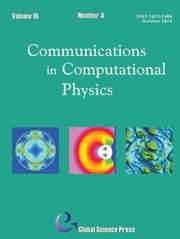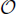Home
Hostname: page-component-55b6f6c457-s8qdg Total loading time: 0.222 Render date: 2021-09-28T02:59:24.180Z Has data issue: true Feature Flags: { "shouldUseShareProductTool": true, "shouldUseHypothesis": true, "isUnsiloEnabled": true, "metricsAbstractViews": false, "figures": true, "newCiteModal": false, "newCitedByModal": true, "newEcommerce": true, "newUsageEvents": true }Communications in Computational Physics

# An Iterative Discontinuous Galerkin Method for Solving the Nonlinear Poisson Boltzmann Equation

Published online by Cambridge University Press:  03 June 2015

*
Corresponding author.Email:hliu@iastate.edu

## Abstract

An iterative discontinuous Galerkin (DG) method is proposed to solve the nonlinear Poisson Boltzmann (PB) equation. We first identify a function space in which the solution of the nonlinear PB equation is iteratively approximated through a series of linear PB equations, while an appropriate initial guess and a suitable iterative parameter are selected so that the solutions of linear PB equations are monotone within the identified solution space. For the spatial discretization we apply the direct discontinuous Galerkin method to those linear PB equations. More precisely, we use one initial guess when the Debye parameter λ =(1), and a special initial guess for λ ≫1 to ensure convergence. The iterative parameter is carefully chosen to guarantee the existence, uniqueness, and convergence of the iteration. In particular, iteration steps can be reduced for a variable iterative parameter. Both one and two-dimensional numerical results are carried out to demonstrate both accuracy and capacity of the iterative DG method for both cases of λ =(1) and λ ≪ 1. The (m + 1)th order of accuracy for L2 and mth order of accuracy for H1 for Pm elements are numerically obtained.

## Keywords

Type
Research Article
Information
Communications in Computational Physics , August 2014 , pp. 491 - 515

## Access options

Get access to the full version of this content by using one of the access options below. (Log in options will check for institutional or personal access. Content may require purchase if you do not have access.)

## References

Holst, M., McCammom, J. A., Yu, Z., Zhou, Y. C., Zhu, Y.Adaptive finite element modeling techniques for the Poisson-Boltzmann equation. Commun. Comput. Phys., 11(1):179214, 2012/01.CrossRefGoogle ScholarPubMed
Debye, P., Hiickel, E.Zur theorie der elektrolyte. Phys. Zeitschr., 24: 185206, 1923.Google Scholar
Kirkwood, J. G.On the theory of strong electrolyte solutions. J. Chem. Phys., 2:767781, 1934.CrossRefGoogle Scholar
Boschitsch, A. H., Fenley, M. O.Hybrid boundary element and finite difference method for solving the nonlinear Poisson-Boltzmann equation. J. Comput. Chem., 25(7):935955, 2004.CrossRefGoogle ScholarPubMed
Baker, N. A., Sept D., D., Joseph, S., Holst, M. J., McCammon, J. A.Electrostatics of nanosystems: application to microtubules and the ribosome. Proc. Natl. Acad. Sci. USA, 98:1003710041,2001.CrossRefGoogle ScholarPubMed
Qiao, Z.-H., Li, Z.-L., Tang, T.A finite difference scheme for solving the nonlinear Poisson-Boltzmann equation modeling charged spheres. J. Comput. Math., 24(3):252264,2006/03.Google Scholar
Colella, Phillip, Dorr, Milo R, Wake, Daniel D. A conservative finite difference method for the numerical solution of plasma fluid equations. J. Comput. Phys., 149(1): 168193, 1999.CrossRefGoogle Scholar
Chen, L., Holst, M. J., Xu, J.The finite element approximation of the nonlinear Poisson-Boltzmann equation. SIAM J. Numer. Anal., 45(6): 22982320, 2007.CrossRefGoogle Scholar
Lu, B., Cheng, X., Huang, J.AFMPB: An adaptive fast multipole Poisson-Boltzmann solver for calculating electrostatics in biomolecular systems. C. Phys. Commun., 181(6):11501160, 2010.CrossRefGoogle ScholarPubMed
Degond, P., Liu, H., Savelief, D., Vignal, M. H.Numerical approximation of the Euler-Poisson-Boltzmann model in the quasineutral limit. J. Sci. Comput., 51: 5986, 2012.CrossRefGoogle Scholar
Liu, H., Yan, J.The Direct Discontinuous Galerkin (DDG) method for diffusion problems. SIAM Journal on Numerical Analysis., 47(1): 675698,2009.CrossRefGoogle Scholar
Liu, H., Yan, J.The Direct Discontinuous Galerkin (DDG) method for diffusion with interface corrections. Commun. Comput. Phys., 8(3): 541564,2010.Google Scholar
Huang, Y., Liu, H., Yi, N.Recovery of normal derivatives from the piecewise L2 projection. J. Comput. Phys., 231(4): 12301243, 2012.CrossRefGoogle Scholar
Oberoi, H., Allewell, N. M.Multigrid solution of the nonlinear Poisson-Boltzmann equation and calculation of titration curves. Biophys J., 65(1): 4855, 1993.CrossRefGoogle ScholarPubMed
Nicholls, A., Honig, B.A rapid finite difference algorithm, utilizing successive overrelaxation to solve the Poisson-Boltzmann equation. Journal of Computational Chemistry, 12(4): 435445, 1991.CrossRefGoogle Scholar
Davis, M. E., McCammon, J. A.Solving the finite difference linearized Poisson-Boltzmann equation: A comparison of relaxation and conjugate gradient methods. Journal of Computational Chemistry, 10(3): 386391, 1989.CrossRefGoogle Scholar
Deng, Y., Chen, G., Ni, W., Zhou, J.Boundary element monotone iteration scheme for semilinear elliptic partial differential equations. Mathematics of Computation, 65(215):943982, 1996.CrossRefGoogle Scholar
Shampine, L. F.Monotone iterations and two-sided convergence. SIAM Journal on Numerical Analysis, 3(4): 607615, 1996.CrossRefGoogle Scholar
Chern, I., Liu, J., Wang, W.Accurate evaluation of electrostatics for macromolecules in solution. Methods and Applications of Analysis, 10(2): 309328, 2003.CrossRefGoogle Scholar
Quarteroni, A., Valli, A.Numerical approximation of partial differential equations. Springer Series in Computational Mathematics, 2008.Google Scholar
Evans, L. C.Partial differential equations. American Mathematics Society, 19, 2010.Google Scholar
Lions, J. L., Magenes, E.Non-Homogeneous Boundary Value Problems and Applications. New-York: Springer-Verlag, 1972.Google Scholar
Liu, H., Yu, H.The entropy satisfying discontinuous Galerkin method for Fokker-Planck equations. Journal on Scientific Computing, accepted (2014).CrossRefGoogle Scholar
Liu, H.Optimal error estimates of the Direct Discontinuous Galerkin method for convection-diffusion equations. Math. Comp., to appear (2014).Google Scholar
4
Cited by

# Send article to Kindle

Note you can select to send to either the @free.kindle.com or @kindle.com variations. ‘@free.kindle.com’ emails are free but can only be sent to your device when it is connected to wi-fi. ‘@kindle.com’ emails can be delivered even when you are not connected to wi-fi, but note that service fees apply.

Find out more about the Kindle Personal Document Service.

An Iterative Discontinuous Galerkin Method for Solving the Nonlinear Poisson Boltzmann Equation
Available formats
×

# Send article to Dropbox

To send this article to your Dropbox account, please select one or more formats and confirm that you agree to abide by our usage policies. If this is the first time you use this feature, you will be asked to authorise Cambridge Core to connect with your <service> account. Find out more about sending content to Dropbox.

An Iterative Discontinuous Galerkin Method for Solving the Nonlinear Poisson Boltzmann Equation
Available formats
×

# Send article to Google Drive

To send this article to your Google Drive account, please select one or more formats and confirm that you agree to abide by our usage policies. If this is the first time you use this feature, you will be asked to authorise Cambridge Core to connect with your <service> account. Find out more about sending content to Google Drive.

An Iterative Discontinuous Galerkin Method for Solving the Nonlinear Poisson Boltzmann Equation
Available formats
×
×